# For P = 140 MPa, determine the range of values of τxy for which the maximum...

For P = 140 MPa, determine the range of values of τxy for which the maximum tensile stress is equal to or less than 60 MPa. (Round the final answer to one decimal place.)

​​​​​​​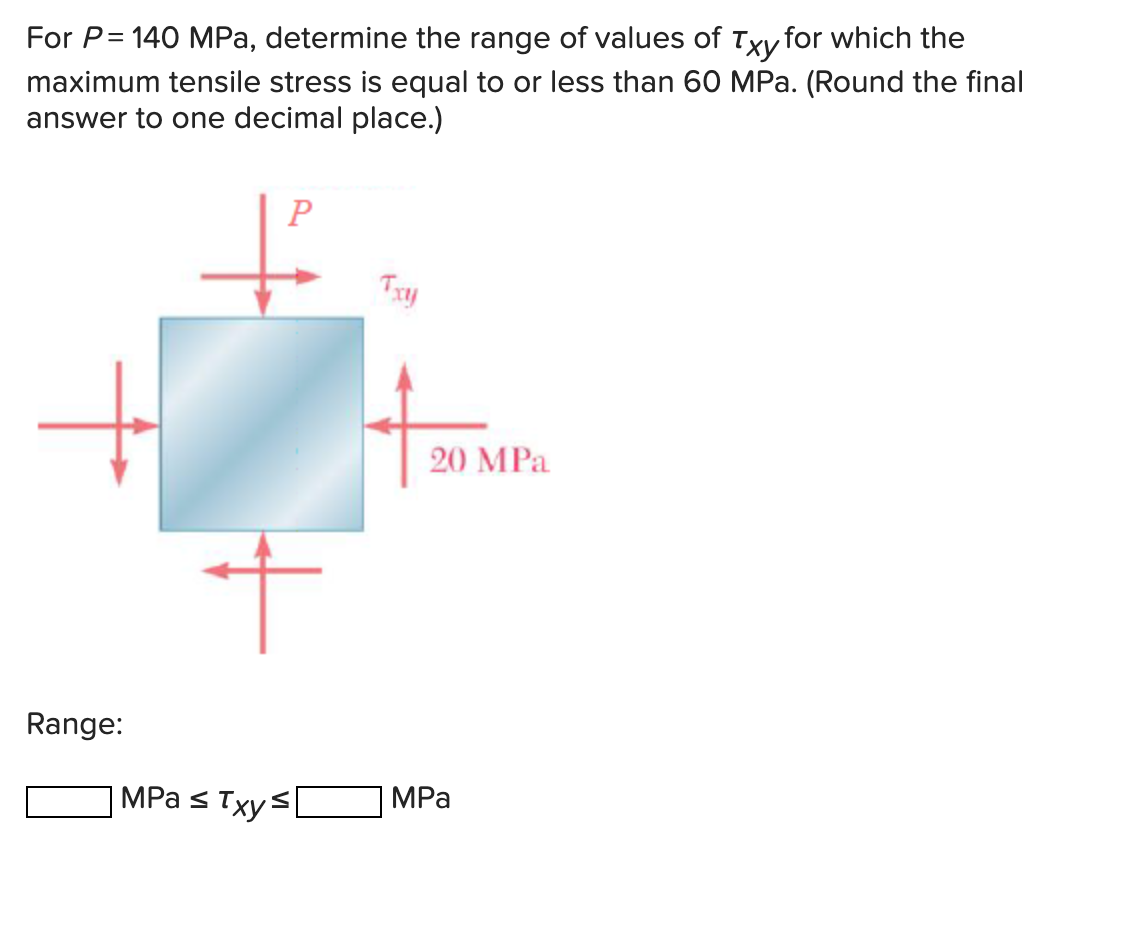For P= 140 MPa, determine the range of values of Txy for which the maximum tensile stress is equal to or less than 60 MPa. (Round the final answer to one decimal place.) 20 MPa Range: OMPa sıxys OMPa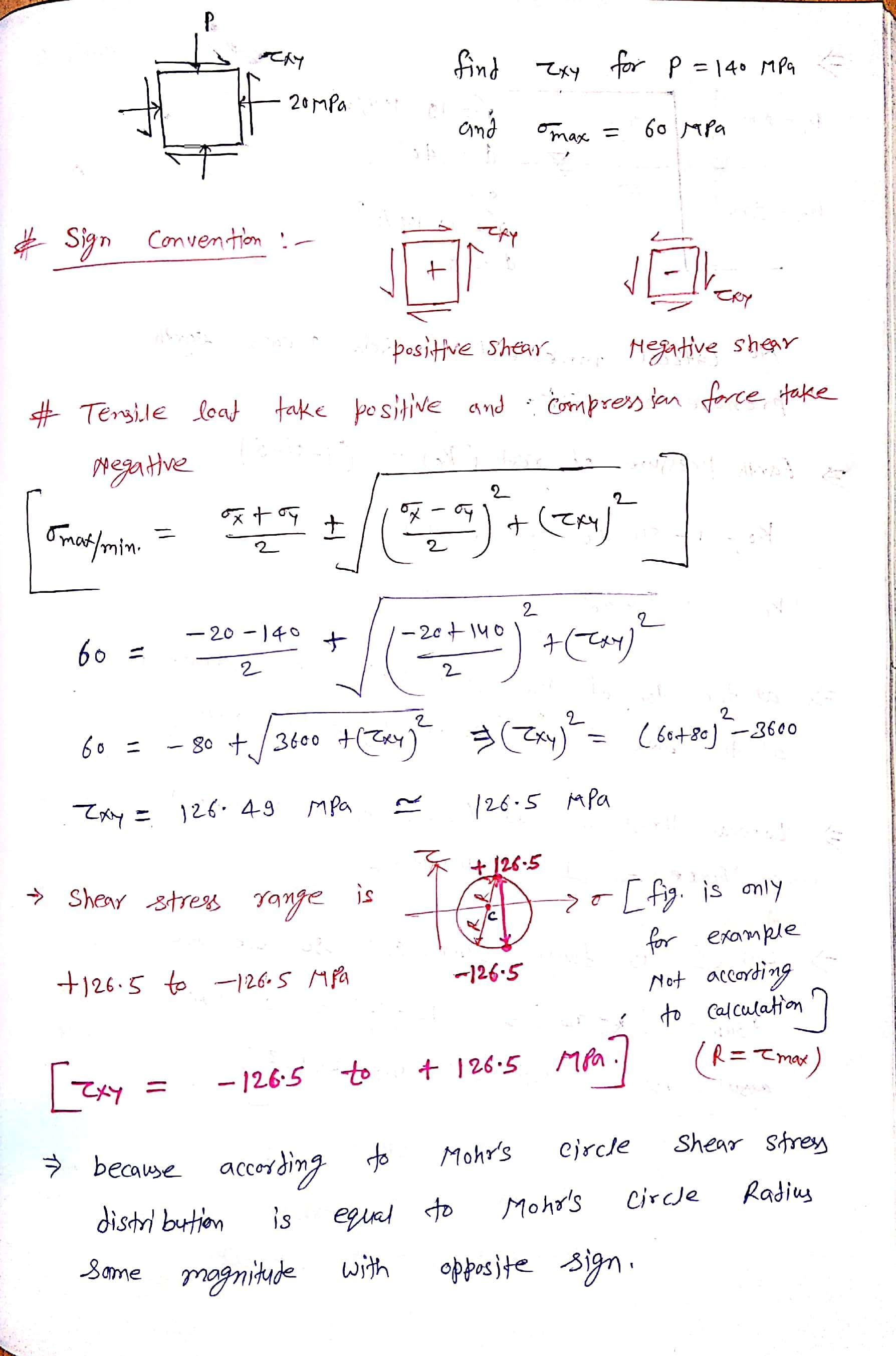##### Add Answer to: For P = 140 MPa, determine the range of values of τxy for which the maximum...
Similar Homework Help Questions
• ### Required information Solve the following problems using Mohr's circle Given: P 84 MPa P 50 MPa...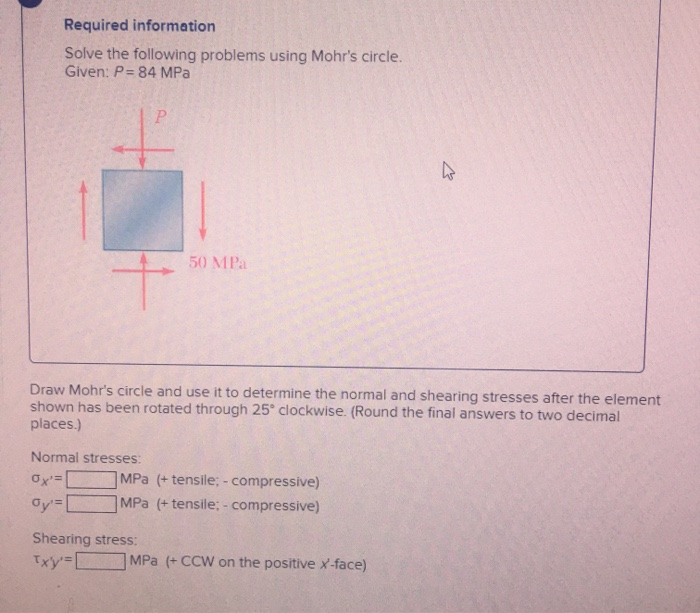Required information Solve the following problems using Mohr's circle Given: P 84 MPa P 50 MPa Draw Mohr's circle and use it to determine the normal and shearing stresses after the element shown has been rotated through 25 clockwise. (Round the final answers to two decimal places.) Normal stresses: MPa (+ tensile; - compressive) Ox' Gy[ MPa (+tensile; - compressive) Shearing stress: Txy MPa (+CCW on the positive X-face)

• ### Chapter 7 Strength of Materials Problem 7.61P7.61 For the element shown,determine the range of values ofTxy for which the maximum tensile stress is equal to orless than 60 MPa.

• ### Determine maximum tensile stress of the beam (Unit: MPa) Set M = 815 N.m; b =25 mm.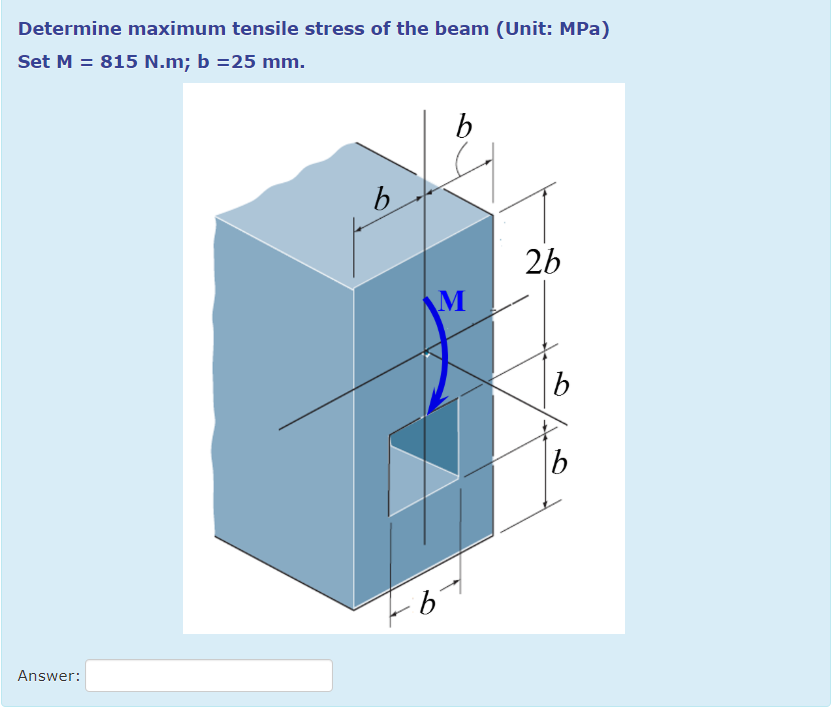Determine maximum tensile stress of the beam (Unit: MPa) Set M = 815 N.m; b =25 mm. Determine maximum tensile stress of the beam (Unit: MPa) Set M 815 N.m; b 25 mm. 2b Answer: Determine maximum tensile stress of the beam (Unit: MPa) Set M 815 N.m; b 25 mm. 2b Answer:

• ### Drawing and using Mohr's circle...Using the state of stress shown, Draw and use Mohr's circleto determine the range of values θ for which themagnitude of maximum shear stress τx'y' is less than or equal to 30 MPa.

• ### A copper strip (Ec 105 GPa) and an aluminum strip (Es 75 GPa) are bonded together...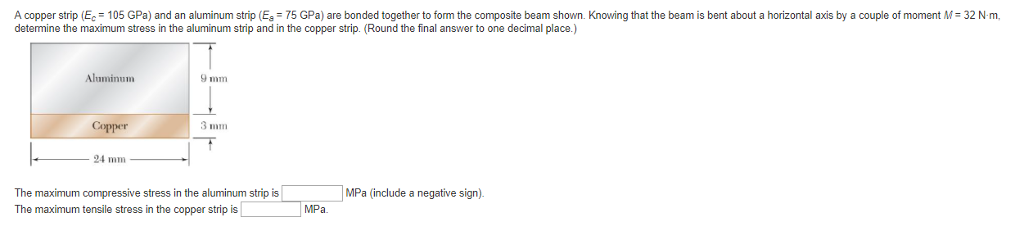A copper strip (Ec 105 GPa) and an aluminum strip (Es 75 GPa) are bonded together to form the composite beam shown. Knowing that the beam is bent about a horizontal axis by a couple of moment M 32 N-m determine the maximum stress in the aluminum strip and in the copper strip. (Round the final answer to one decimal place.) Aluminum 9 mm Copper 3 mm 24 mm The maximum compressive stress in the aluminum strip is The maximum...

• ### Using an allowable stress of 154 MPa, determine the largest bending moment M that can be...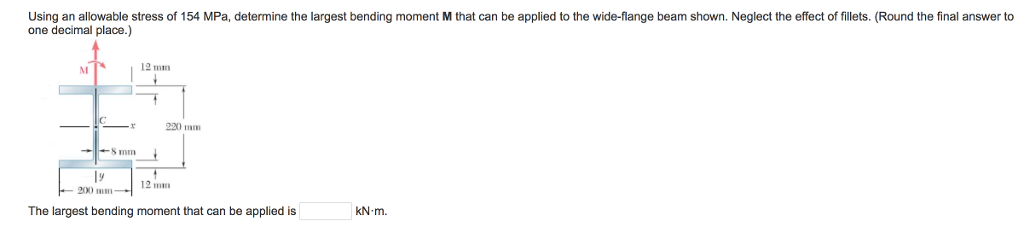Using an allowable stress of 154 MPa, determine the largest bending moment M that can be applied to the wide-flange beam shown. Neglect the effect of fillets. (Round the final answer to one decimal place.) 12 mm 12 mm 200 mm The largest bending moment that can be applied is KN-m.

• ### The grain of a wooden member forms an angle 15° with the vertical. For Txy =...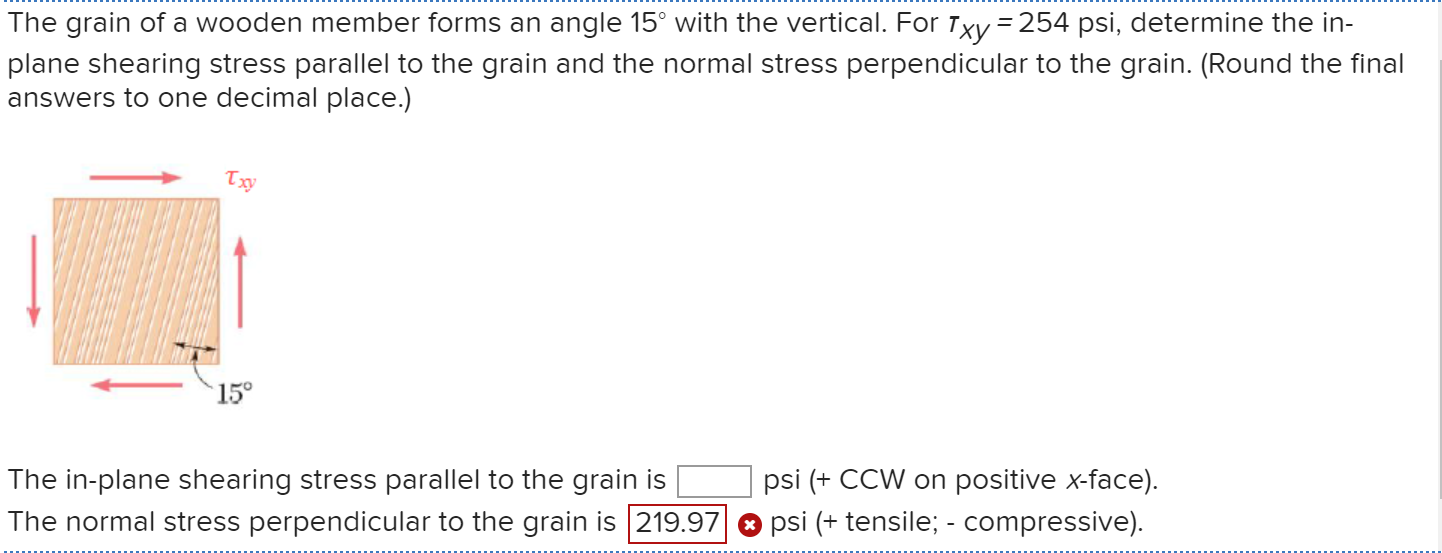The grain of a wooden member forms an angle 15° with the vertical. For Txy = 254 psi, determine the in- plane shearing stress parallel to the grain and the normal stress perpendicular to the grain. (Round the final answers to one decimal place.) Ty The in-plane shearing stress parallel to the grain is The normal stress perpendicular to the grain is 219.97/ psi (+ CCW on positive x-face). psi (+ tensile; - compressive).

• ### Props. 4-0 //00 4-89. The steel bar has the dimensions shown. Determine the maximum axial force...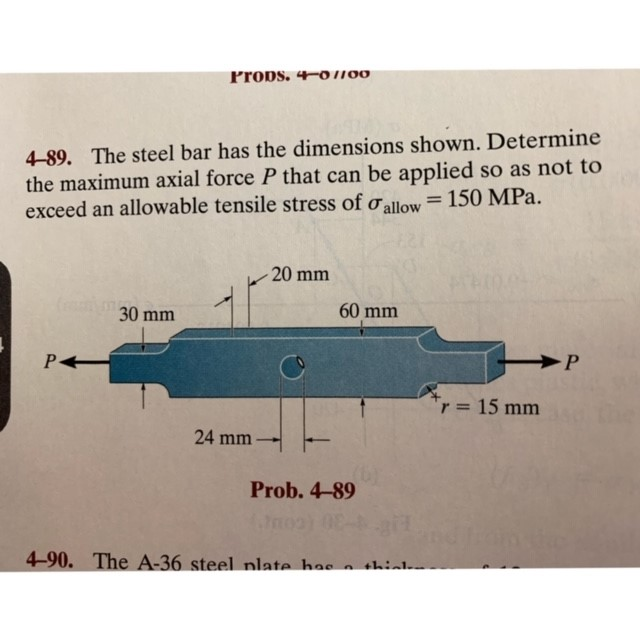Props. 4-0 //00 4-89. The steel bar has the dimensions shown. Determine the maximum axial force P that can be applied so as not to exceed an allowable tensile stress of oallow= 150 MPa 20 mm 60 mm 30 mm P< r=15 mm 24 mm Prob. 4-89 4-90. The A-36 steel nlate has

• ### Problem 1 A thin-walled pressure vessel of mean radius R 100 mm and thickness t (R) is subjected to cyclic internal pressure p in the range -3 MPa p7 MPa. Using Soderberg's relation with maxim...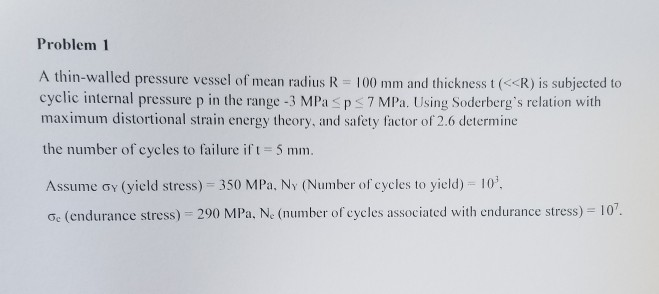Problem 1 A thin-walled pressure vessel of mean radius R 100 mm and thickness t (R) is subjected to cyclic internal pressure p in the range -3 MPa p7 MPa. Using Soderberg's relation with maximum distortional strain energy theory, and safety factor of 2.6 determine the number of cycles to failure ift-5 mm Assume ơy(yield stress)-350 MPa, NY (Number of cycles to yield)-10, ơ (endurance stress)-290 MPa, Ne (number ofcycles associated with endurance stress)-10 Problem 1 A thin-walled pressure vessel...

• ### Question 4 O out of 12.5 points X Determine the maximum shear stress in MPa) at...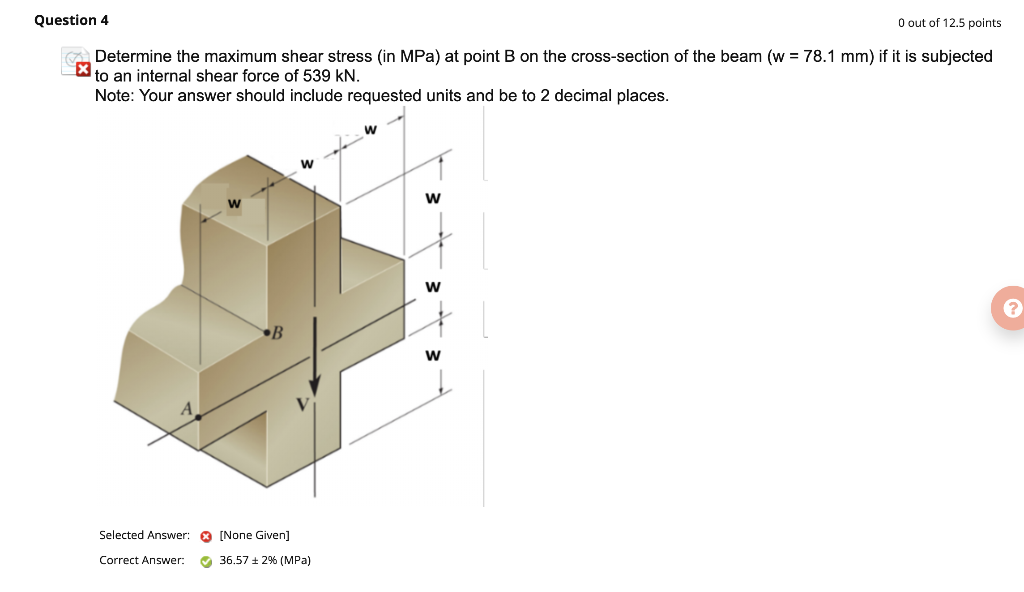Question 4 O out of 12.5 points X Determine the maximum shear stress in MPa) at point B on the cross-section of the beam (w = 78.1 mm) if it is subjected to an internal shear force of 539 KN. Note: Your answer should include requested units and be to 2 decimal places. w w w ? B w Selected Answer: ® [None Given] Correct Answer: 36.57 + 2% (MPa) Question 5 O out of 12.5 points X The bar...

Free Homework App